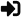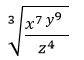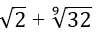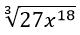•• X

to continue to ilmkidunya.com

X

to continue to ilmkidunya.com

X

### Register Type

Please Provide following information to Register

• Student
• Tutor
• Consultant
• Employer
×

General math is an important and technical subject of 9th class arts students. Some students find it tough and hard as they could not grasp its different formulas and tricky concepts. General maths is a subject that can be made easy by doing more practice. For getting maximum marks in general math, 9th students should make maximum practice of this. We are providing to you the best way for getting good marks in general maths on Ilmkidunya. 9th class general math guess paper 2021 is available here for all students of 9th class.

## General Maths Guess Papers of 9thClass

9th class students can get well prepared for board exam by preparing general math guess papers of 9. Our experienced staff made these important guess papers after collecting important material from different sources on experience basis for the better preparation of 9th class students. We are provided to you these important guess papers here for free. Ilmkidunya is providing you the best way for getting the good marks in general maths. Download these advantageous and important guess papers of general maths and get ready for better performance in the general maths board exam 2021. 9th class General math guess paper 2021 is given here.

ACCELERATED LEARNING PROGRAMME (ALP)

LAHORE, SAHIWAL, GUJRANWALA, FAISALABAD, MULTAN, BHAWALPUR

RAWALPINDI , DERA GHAZI KHAN, AZAD KAHSMIR , SARGODHA

9TH CLASS GENERAL MATHEMATICS (ARTS GROUP)

UNIT NO.1 PERCENTAGE, RATIO AND PROPORTION.

1. EXERCISE NO. 1.1
1. Q.1 (i)
2. Q.2 (i) ,(v)
3. Q.4 (vii)
2. EXERCISE NO.1.2 Q.1, 2,3,4,5,7, 9
1. EXAMPLE -2 1.1.3
2. EXERCISE NO. 1.4 Q.1 , 2, 3, 7, 8
3. EXERCISE -1.5 Q.1,2,3,5,7,8,9,10

UNIT NO. 2 ZAKAT, USHR AND INHERITANCE.

1. EXERCISE NO. 2.1
1. Q.1
2. Q.2
3. Q.3
4. Q.4
5. Q.5
6. Q.6
7. Q.7
8. Q.10
9. Q.11
10. Q. 12

1. EXERCISE NO. 3.1
1. Q.1 (i) , (vi)
2. Q.4
3. Q.5
4. Q.3 (i) , (iv) , (v)
2. EXERCISE NO. 3.2 Q.1 (i)
1. Q.5
2. Q.6
3. Q.2 (ii)
3. EXERCISE NO. 3.3
1. Q.1
2. Q.2
3. Q.3

UNIT NO. 4 FINANCIAL MATHEMATICS.

1. EXERCISE NO. 4.1
1. Q.1
2. Q.2
2. EXERCIS NO. 4.2
1. Q.1
2. Q.2
3. Q.3
4. Q.6
3. EXERCISE NO. 4.3
1. Q.6
2. Q.7
3. Q.8
4. Q.9

UNIT NO. 5 CONSUMER MATHEMATICS.

1. EXERCISE NO. 5.1
1. Q. 1
2. Q.5
3. Q.6
4. Q.7
2. EXERCISE NO. 5.3
1. Q. 2
2. Q. 4
3. Q.5
4. Q. 7

UNIT NO. 6 EXPENSENTS AND LOGARITHMS.

1. EXERCISE NO. 6.1
1. Q.3 (iii)
2. Q.4 (x)
3. Q.5 (iii)
2. EXERCISE NO.6.2
1. Q.28 (v)
2. Q.28
3. Q.29
3. EXERCISE NO.6.3
1. Q.1 1 , 3
2. Q.11
3. Q.12
4. Q.13
5. Q.14
6. Q.15
4. EXERCISE NO. 6.4
1. Q.4 (ii) Q.3
2. Q.4
3. Q.5
4. Q.6
5. EXERCISE NO.6.5
1. Q.1 (iii)
2. Q.2 (i)
3. Q.3
4. Q.4
5. Q.7
6. Q.9
7. Q.10
8. Q.11

UNIT NO. 7 ARITHMETICS AND GEOMETRIC SEQUENCE.

1. EXERCISE NO. 7.1
1. Q.1 (i) , (iii), (iv) , (vii)
2. EXERCISE NO. 7.2
1. Q.1 (i),(iv)
2. Q.2 (i), (v)
3. Q.4
4. Q.10
3. EXERCISE NO. 7.3
1. Q.1 (i) , (ii), (iii) , (iv)
2. Q.2
3. Q.3
4. Q.4
5. Q.5
6. Q.9
4. EXERCISE NO.7.5
1. Q.1 (i) , (ii)
2. Q.2 (i) , (ii)

UNIT NO. 8 SET AND FUNCTIONS

1. EXPERCISE NO. 8.1
1. Q.1
2. Q.2
3. Q.3
4. Q.4
5. Q.5
6. Q.6
7. Q.7
2. EXERCISE NO. 8.2 Q.1
1. Q.2
2. Q.3
3. Q.4
4. Q.5

UNIT NO. 9 LINEAR GRAPHS

1. EXERCISE NO. 9.1
1. Q.1 (vi)
2. Q.3 (i) , (ii), (iv)
2. EXERCISE NO. 9.3
1. Q.1 (b)
2. Q.3

UNIT NO. 10 BASIC STATISTICS

1. REVIEW EXERCISE. Q.3
1. Q.5
2. Q.6

OR

SHORT QUESITONS:

• Express 95% as fractions in lowest form.
• Express the decimal 0.065 as percentage.
• If there are 800 cars in a car parking and 80% of them are Pakistan made. Find the number of Pakistani cars.
• Simplify the ration 24:12 in the simplest form
• Express 12/10 : 28/10 in its simple form
• What is meant by cost price and sale price?
• Define direct proportion.
• Find the ratio of Rs. 160 per meter to Rs.150 per meter.
• Express ratio 2/3,3/5 in its simplest form.
• Find the unknown ‘x’ in the proportion x : 3 :: 60 :15
• Calculate Zakat on Gold amounting to Rs. 11, 1,000/-
• Calculate the amount of Zakat on an amount of Rs. 5000000.
• Find CP when SP = Rs.572/- and profit is 5%
• Find the SP when CP= Rs. 1540/- Loss = 5%
• Find the marked Price when SP = Rs. 2400, Discount = 4%
• Find CP when SP = Rs.851 and loss = 5%
• Convert 700 Saudi Riyal into Pakistan rupees. When the rate of Saudi Riyal = Rs.22, 400.
• Convert 250 US dollars into Sterling pound.
• Define excise duty
• The annual income of a flat is Rs. 14, 00,000. Find the tax payable at the rate of 16%
• Define Sales tax.
• A computer price is Rs. 34800 inclusive of 16% sales tax what is the original price of the computer.
• Simplify (x2y3)1/6
• Write 0.00018 in scientific notation.
• Solve the equation Log, (x+1) = 2
• Write down the value of log 52.13
• Express in exponential form:•simplify it.
•Simplify it.
• Find A.M between 4 and 8
• Find G.M. between 4 and 9
• Find the 5th term of a G.P. 4, -12, 36………..
• Convert 20 oC into oF.
• Find the range of the given data 10,15,9,5, 22
• Find the standard division of the values 2,3,6,8,11

LONG QUESTIONS:

• Amina scored 45 out of 50 in a math test, 64 out of 75 in chemistry test and 72 out of 80 in a physics test. In which subject did she perform best?
• A shop keeper plans to produce 200 articles with the help for 5 persons working 8 hours daily. How many articles can be made by 8 persons if they work 5 hours daily.
• The price of 20 pens is Rs.2000. What will be the price of 40 such pens?
• Calculate zakat on gold of worth Rs. 8, 00,000, cash of amount Rs. 4, 00,000 and silver of weight 50 tola (Rs.5000 per tola).
• If 15% discount on MP of a heater is allowed and still makes a profit of 2%. If it is sold on MP, what is profit percentage?
• Find compound profit on Rs.800 for 4 years @ 6 percent per annum.
• Evaluate. 8.67 x 3.94/1.78
• Draw the graph of y = 3x
• Distribute Rs. 33,000 as a profit in a business regarding three persons if their shares are in the ratio 3: 5:3
• Insert two G.M. between 4 and 1/2

## 9th Class General Math Guess Papers 2021

In these guess papers, we are providing you those possible questions which can be asked in your general math board exam. These important guess papers of 9th class general maths are made according to your Punjab textbook board. These guess papers are well designed and well represented just like your board exam. You can easily download 9th class general math guess paper in pdf for the best preparation.